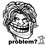# I Can't....Can Anybody else?

I have two problems-

1.Find all primes $$p$$ such that there are positive integers $$a$$ and $$b$$ for which $$p=a^{2}+b^{2}$$ and $$a^{2}+b^{2}$$ divides $$a^{3}+b^{3}-4$$.

2.Find all natural numbers $n$ and $k$ such that $2^{n}+3=11^{k}$.Note by Souryajit Roy
5 years, 9 months ago

This discussion board is a place to discuss our Daily Challenges and the math and science related to those challenges. Explanations are more than just a solution — they should explain the steps and thinking strategies that you used to obtain the solution. Comments should further the discussion of math and science.

When posting on Brilliant:

• Use the emojis to react to an explanation, whether you're congratulating a job well done , or just really confused .
• Ask specific questions about the challenge or the steps in somebody's explanation. Well-posed questions can add a lot to the discussion, but posting "I don't understand!" doesn't help anyone.
• Try to contribute something new to the discussion, whether it is an extension, generalization or other idea related to the challenge.

MarkdownAppears as
*italics* or _italics_ italics
**bold** or __bold__ bold
- bulleted- list
• bulleted
• list
1. numbered2. list
1. numbered
2. list
Note: you must add a full line of space before and after lists for them to show up correctly
paragraph 1paragraph 2

paragraph 1

paragraph 2

[example link](https://brilliant.org)example link
> This is a quote
This is a quote
    # I indented these lines
# 4 spaces, and now they show
# up as a code block.

print "hello world"
# I indented these lines
# 4 spaces, and now they show
# up as a code block.

print "hello world"
MathAppears as
Remember to wrap math in $$ ... $$ or $ ... $ to ensure proper formatting.
2 \times 3 $2 \times 3$
2^{34} $2^{34}$
a_{i-1} $a_{i-1}$
\frac{2}{3} $\frac{2}{3}$
\sqrt{2} $\sqrt{2}$
\sum_{i=1}^3 $\sum_{i=1}^3$
\sin \theta $\sin \theta$
\boxed{123} $\boxed{123}$

Sort by:

@Souryajit Roy If you can't, no one can ;)

- 5 years, 9 months ago

Sweet Sarcasm

- 5 years, 8 months ago

Hey @Souryajit Roy , I got the answer for problem 2. The only solution is $(3,1)$. I will post the solution soon. It's very easy.

- 5 years, 9 months ago

When you will give the solution ? It has been a month -_-

- 5 years, 7 months ago

- 5 years, 7 months ago

Finally I solved it...no longer in need of a solution

- 5 years, 7 months ago

Can you post the solution here? Plss

- 5 years, 7 months ago

Sorry, I lost the paper on which I wrote the solution. And I forgot the way I proved it as it is too long. Sorry :(

- 5 years, 7 months ago